V1

2023/03/21阅读：39主题：橙心

# 拉丁方设计资料与两阶段交叉设计资料的方差分析

## 拉丁方设计

### 拉丁方设计注意事项:

A)三个因素中有一个是关注因素，另外两个是非关注因素。

B) 三个因素的水平数必须相同，以关注因素的水平数为准。

C)三个因素之前不存在交互作用或交互作用可以忽略。

### 设计步骤

A）根据因素的水平数选定拉丁方表。

B）将选定拉丁方表随机化，即行、列交换。

C）规定行、列、字母代表的因素和水平

### 变异分解

SS总 = SS处理 + SS行区组 + SS列区组 + SS误差

### 举例：

H0：六种药物组间，家兔间，注射部位间的总体均数相等。

H1：六种药物组间，家兔间，注射部位间的总体均数不等或不全相等。

α=0.05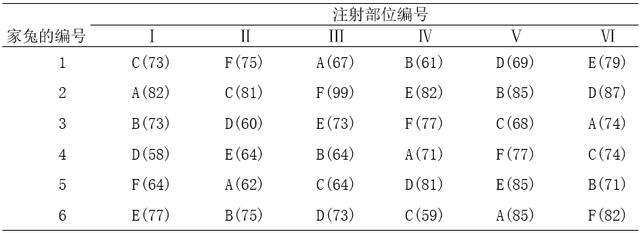### 代码演示

``#拉丁方设计资料的方差分析library(readxl)#载入数据df <- read_xls("E04_05.xls")dfout <- aov(Results~ factor(Group) + factor(ID) + factor(Treat), data = df)res <- rstandard(out) #提取标准化残差赋值给respre <- predict(out) #提取预测值赋值给premydata <- data.frame(df, res, pre) #重新形成数据集View(mydata)  #查看数据``
##### 检验方差齐性
``#方差齐性检验leveneTest(Results ~ factor(Treat), data = mydata, center = mean)#方差齐性检验summary(aov(res ~ factor(Treat), data = mydata))#F检验残差差异``

##### 正态性检验
``#正态性检验shapiro.test(mydata\$res)``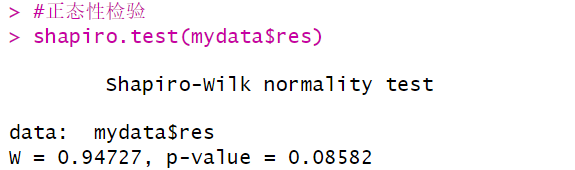数据满足正态分布。

##### 差异性分析
``out <- aov(Results~ factor(Group) + factor(ID) + factor(Treat), data = df)summary(out)plot(TukeyHSD(out))#两两比较``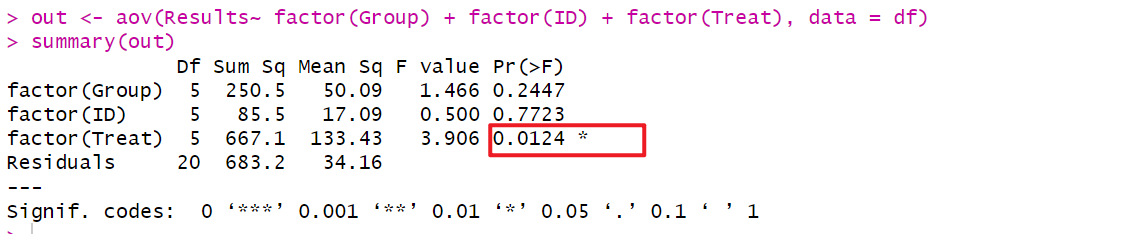结论：还不能认为6只家兔皮肤疱疹大小总体均数不全相等；不能认为6个注射部位皮肤疱疹大小总体均数不全相等；6种药物注射后家兔产生的皮肤疱疹大小总体均数不全相等（P<0.05）。

## 两阶段交叉设计

### 举例：

#### 变异分解

SS总 = SS药物处理 + SS阶段 + SS受试者 + SS误差

### 代码演示

``library(readxl)df2 <- read_xls("E04_06.xls")df2``
``AOV <- aov(y ~ factor(treat) + factor(person) + factor(phase), df2) #计算方差分析模型res <- rstandard(AOV) #提取标准化残差赋值给respre <- predict(AOV) #提取预测值赋值给premydata <- data.frame(df2, res, pre) #重新形成数据集View(mydata)  #查看数据``
``#生成模型残差，并分析方差齐性library(car)leveneTest(y ~ factor(treat), data = mydata, center = mean)#方差齐性检验t.test(res ~ factor(treat), data = mydata)#T检验残差差异``

``shapiro.test(mydata\$res)#正态性检验``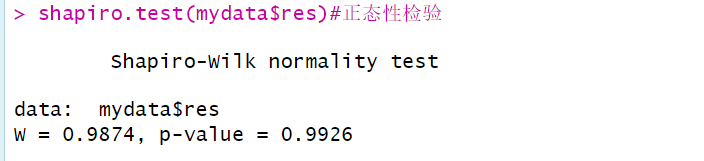正态性检验结果显示P=0.9926>0.05，提示因变量残差近似服从正态分布。

``#差异分析summary(AOV)``
``#两两比较plot(TukeyHSD(AOV))``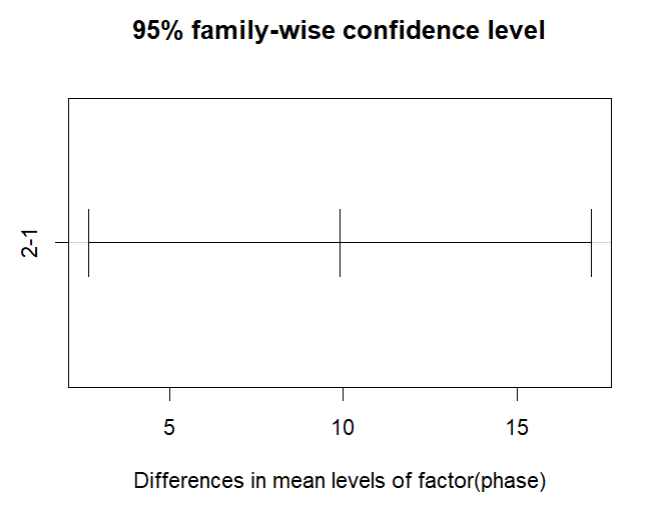结论:
1.还不能认为 A 和 B 两种闪烁液的测定结果有差别。
2.可认为测定阶段对测定结果有影响。
3.可认为各受试者的H-cGMP 值不同。

### 参考资料



《医学统计学》[第五版]: 孙振球，徐勇勇，人民卫生出版社



《医学统计学》[第五版]: 孙振球，徐勇勇，人民卫生出版社

PythonV1